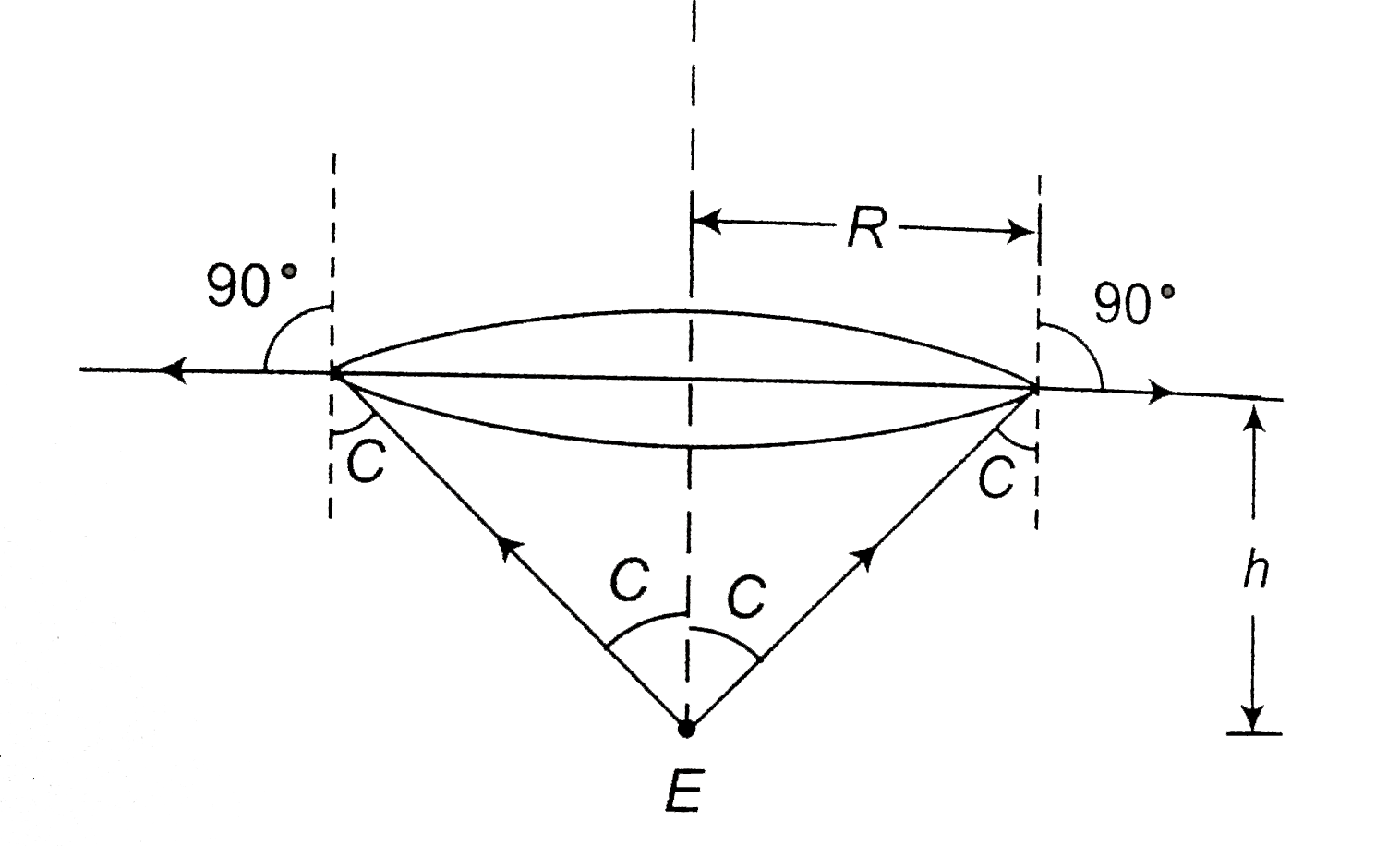# An air masked eye placed inside water refractive index, mu sees the outside world. The vertex angle of the cone in which the eye will see the outsid

114 views
in Physics
closed
An air masked eye placed inside water refractive index, mu sees the outside world. The vertex angle of the cone in which the eye will see the outside world is
A. 2sin^-1(1//mu)
B. 2cos^-1(1//mu)
C. 2sin^-1sqrt((mu^2-1))
D. 2cos^-1sqrt((mu^2-1))

by (84.0k points)
selectedmusinC=1xxsin90^@
sinC=1//muimpliesC=sin^-1(1//mu)
Vertex angle =2C=2sin^-1(1//mu)